# Tutorial on Cloth Modelling

by

 P S Karthikeyan P S Ranganathan III year, B.Tech IV year, B.Tech Aerospace Engineering Computer Science Engineering Indian Institute of Technology Sri Venkateswara College of Engg. Madras Pennalur, Madras

for

ACM Student Tutorial Contest

Address all correspondence to

P S Karthikeyan
74/10 Gem Flats,
Anna Nagar West Extension,
e-mail: [email protected]

# Tutorial on Cloth Modelling

This tutorial deals with modelling cloth from the perspective of displaying the cloth in different situations like cloth draped over a solid object, cloth draped on a mannequin, freely hanging cloth, cloth flowing in air etc. We will give a general overview of different methods that have been developed so far. We will deal with one method in detail and guide you through writing your own simulations using that model.

## Introduction

Efforts to model the complex creases and drapes of cloth can be classified under two different groups.
 Computer graphics animators who have been engrossed only with the draping and shape of cloth from an animators point of view for the sheer pleasure of animating a natural phenomena. They wanted to produce models which exhibit cloth like behaviour. They were not interested in lending a physical meaning to their work. The models could not be used to predict how different cloth will behave. It would generate results for a generic cloth. CAD Engineers who wanted to model cloth in terms of its mechanical behaviour. They treated it as an exclusive problem of structural mechanics. They were interested in measuring traditional mechanical properties like Young's modulus, Poisson ratio etc. These are utterly inadequate for predicting the drape of fabric. They did not consider the internal structure of cloth as a weave of threads. We will not be dealing with these models in this tutorial. Some work has been done incorporating the above two approaches. This was mainly inspired by apparel designers, interior designers etc who were interested in the behaviour of different types of cloth which was part of their professional work. For example an interior designer would like to know how a woollen curtain would hang compared to a cotton one.

In this tutorial we will give you an overview of work done by people using method 1 and give an exhaustive explanation of the work done by David Breen et al and Eberhardt et al. among others using method 3. After reading this tutorial you will be in a position to write code to model the draping behaviour of cloth. We will not be dealing with method 2 which is mainly structural mechanics.

## Computer Animation Models

Computer animators modelling cloth were not concerned with the intrinsic properties of cloth specific to a type of cloth. All they attempted was what a "cloth like material" or "average cloth" would behave under whatever constraints they were modelling. For example rendering the shape of a square peice of cloth when it is draped on a sphere.

This approach itself can be broken down into 3 parts.

• Goemetric modelling The cloth is not physically modelled as a new structure. We extend other mechanics theories empirically. These methods were computationally very fast but visually not very appealing.

• Physical modelling using energies The cloth is considered as a new structure for which a model is suggested. These methods were basically energy minimization models. They were based on the law of nature that

Every body tends to an equillibrium position in which it has its minimum energy.

Every body has potential energy and kinetic energy. Kinetic energy as we all know is due to its velocity given by (mv2)/2. Potential energy of cloth is what we dont know. This is defined differently according to model chosen.

Once we have the potential energy and kinetic energy our problem is half solved. All we have to do is define the cloth using a particle grid to discretise the problem. Then use the Lagrange equation of motion: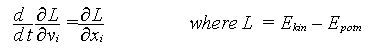The Lagrange equation is a fundamental equation of kinematics, which can be used to determine equillibrium position when the energy of a body is known. Physically is states that:

The rate of change of (rate of change of Lagrangian with velocity) is effectively the rate of change of Lagragian with space. The Lagrangian is (Kinetic energy) - (Potential energy). This is true for each dimension. This is what is expressed by the above equation. To read more about the Lagrangian look in any standard Mechanics book.

We need to discretise the problem to solve this differential equation. We further need the boundary condition to solve the problem fully. The boundary conditions for these problems will be:

1. At time t = 0, the cloth is a rest. The velocity of all points is zero.
2. We can arbitrarily define a spatial boundary condition giving the location of all points at t = 0. For example if we are draping the cloth on a cube, we obviously start with the cloth resting like a sheet on the cube and then allow it fall and drape itself. See figure below.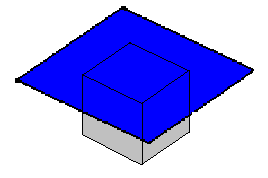This gives a further set of i * j conditions.

Using these boundary conditions and solving the differential eqautions numerically will give us a set of simultaneous equations involving the postions of the elements of the grid (i,j) for all point of the grid. Solving these equations will give equillibrium position of the grid points, which amounts to the drape of the cloth.

• Physical modelling using Forces The physical model can also be attempted using forces. Then we use the law :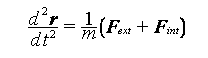where r is the position vector of a point. We determine all forces (internal and external) that are acting on the cloth. Discretise the problem and then solve the set of simultaneous equations to give the position of particles at equllibrium similar to what was done in the previous method.

## Geometric Models

Geometric models do not consider the physical properties of cloth. Rather, they focus on the appearance, particularly folds and creases, which they represent by geometrical equations. Geometrical techniques require a considerable degree of user intervention. They are highly specific solutions. For example one technique can represent cloth hung from a fixed number of points very well. Another can represent creases and folds very well.

### The work of Weil

Weil1 was probably the first person to model cloth in any method whatsoever. He did this work in 1986 using a very simple geometric model extended from the theory of cables. He worked on the specific problem of cloth hanging from a finite number of points (say 3 or 4).

A cable under its self-weight forms a catenary curve at equillibrium position. A catenary curve has the equation

y = a cosh(x/b)

We can extend this logic to 3D for a cloth. A cloth hanging under constraints at finite number of points is a logical extension of a catenary curve in 2D. So we can develop a system of catenary curves to represent the cloth.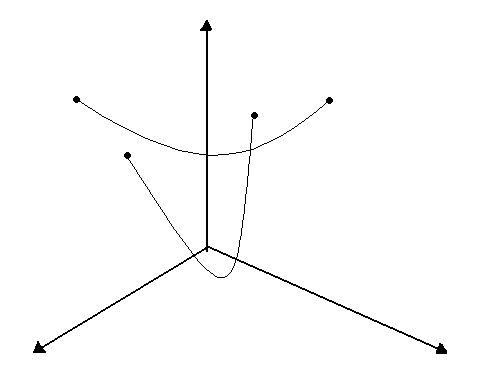If we have cloth hanging at 4 points. Then we fit catenary curves between the 2 diagonals. These two will naturally intersect. What we have to do is that find a resultant curve, but to simplify matters we will just ignore the lower of the two curves.

At this stage we have a wireframe describing the rough shape of the cloth. We further refine the shape by dividing the cloth into smaller and smaller triangles and fitting catenary curves among this. This will generate a better and better picture. We can stop at any arbitrary point depending on the accuracy of the simulated shape we require.

### Another geometric method

This method is being presented for the first time in this tutorial. It has been developed by none other than Yours Truly. It is similar to the Weil method in the sense that it is also derived from the theory of cables. A working simulation has been developed. Look in the Appendix.

The method is meant to simulate a polygonal peice being hung at each vertex at any height. Look at figure below.What we have done is extended the logic of painting a polygon to rendering its shape in 3D. To make explanations easier let us consider a cloth which is in the shape of a quadrilateral.

Essentially what we are doing while rendering hanging cloth is finding the different heights which different points of the cloth obtain at equillibrium. We consider a quadrilateral connecting the 4 points. Then consider a square encompassing the quadrilateral as shown in figure above. The square is divided into a finite number of cells. We consider only the points which fall inside the quadrilateral. At the boundaries we take the point closest to the line directly connecting the vertices concerned.

We have effectively discretised the problem. All we have to do now is find the height of all points in our grid which lie inside the quadrilateral.

The first step is to find the shape at the boundaries, because this is the easiest to guess.

What is the most likely shape that the cloth takes between two vertices. It is most probably a catenary. The reasons why we can choose this are:

1. Cloth is light and the vertices are not much influenced by the weight of the cloth at other vertices.
2. Using assumption 1, between two vertices if we can assume the only effective force acting on the cloth are weight of the cloth around and between the vertices. This can be safely approximated to a cable. Even if the approximation is wrong, we are only drawing the border of the cloth as a cable.

We extend this logic to all the vertices of the polygon. So now we have a set of catenary curves which give the boundary of the cloth. We have to determine the shape of the intermediate cloth.

The problem has now been reduced to finding a route for curve AB to CD with boundary condition AD and BC at the edges for all points. This can be considered as averaging the effect of the 4 boundary conditions for all points.

Each interior point is influenced only by its neighbouring 4 points as far as its height is concerned ie we can assume each point to be held up by its neighbouring points which can be seen from the weave of cloth. Therefore we can deduce that

Hi,j = { Hi,j-1 + Hi,j+1 + Hi-1,j + Hi+1,j } / 4, where Hi,j is the height of the point at (i,j).

Using the boundary condition a first approximation for the location of all points can be derived. We keep iterating until a stable configuration is reached. This can be defined as

abs ( Hi,jk - Hi,jk+1 ) <= minvalue,

where minvalue can be defined according to precision required.

Now we have the location of all points. To render the shape of cloth we fit a polygon between 4 points and raytrace the scene.

Advantages of this method are:

1. Speed of rendering
2. Cloth which is textured (ie has a pattern on it can also be rendered)
3. Computationally cheap in terms of memory and hardware
4. After computing the surface, rendering can been done in any freely available 3D modeller like POV ray, 3D studio etc.

Drawbacks of the method:

1. We totally ignore the fact that the cloth stretches.
2. The assumptions are purely empirical and are not proved, although by physical reasoning we can say they are plausible assumptions.

The logic of the cloth taking a catenary between 2 vertices can be applied to any and every pair of vertices of the cloth. So we can generate actually 6 curves for our quadrilateral. Plugging all six initial conditions before we begin our iteration will converge to the solution faster than using only 4.

But while using six conditions what do we do at the point of intersection of the diagonals, because we will get 2 different heights for both diagonals? We take either the average, or a weighted average depending on the perpendicular distance of the diagonals from the center of mass of the cloth.

While fixing the catenary curve between 2 vertices we use the equation of the catenary

y = acosh ( x + b)

We have two points and two constants a, b. Using the two points we evaluate the two points a, b.

In the simulation we have simulated a square peice of cloth hanging from the ceiling. So our catenary reduces to y  =  a  cosh  (x)  for all 4 edges. We then fill in the inside points. We have developed our own 3D viewer to view the model. The image of the cloth obtained is included.

## Physical Models

In physical models various structural studies are done on the cloth and its intrinsic behaviour is attempted to be simulated. This basically involves finding the potential energy of the cloth, because in cloth the weaves are held just by the friction and tension amongst the threads. Various methods and models have been proposed since Weil's first geometric model. We present below a brief overview of some of the methods.

1. The work of C Feynman2 - He represented the cloth by a 2D grid and the final postion of cloth was derived based on the minimization of energy. The tendency of any body in nature is to reduce its energy to a minimum. So we calculate the enery of all points and minimize it to get the final position of the cloth. The Energy equation he took was of the form

### E(Pi,j) = ksEelastic(i,j) + kbEbending(i,j) + kgEgravitational(i,j)

where ks, kb, ks are the elasticity, bending and density constants. The energy at any point (i, j) is calculated relative to the surrounding 8 points. The figure below explains the formation of the grid from cloth. The figure on the left if the cloth and the one on the right is the grid. P(i,j) contains position, velocity, acceleration and all other data about that point. The energy minimization was done using the method of steepest descent.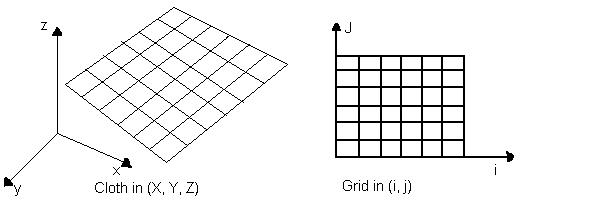Do not confuse this Feynman with the illustrious Richard Feynman who passed away recently!

2. The work of Terzopoulos3 - Terzopoulos introduced a deformable model intended for generalized flexible objects, but the model has been used in several cases for cloth visualisation. The figure below represents his model.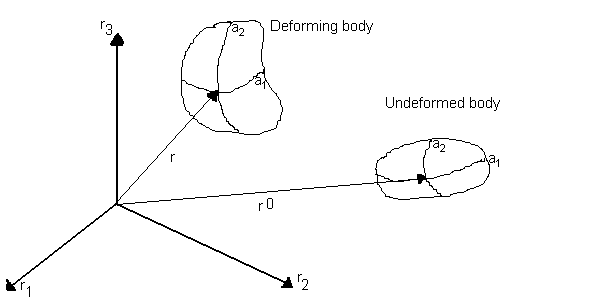This model did not take into consideration the weave of the cloth ie changes of properties in the weft and warp directions. He considered just one single internal elastic force.

The exact physical model is explained below. The reduction of the generalised model to cloth is too mathematical and the whole result of the operation is to setup a finite difference scheme which reduces to a set of simultaneous ordinary differential equations. You can skip the section below if you are frightened by the math.

The position of a general point a is

r(a, t) = [r1(a, t), r2(a, t), r3(a, t) ]
Referring to the figure above. The body in its undeformed state can be expressed by the vector below
r0(a, t) = [r01(a), r02(a), r03(a) ]

Lagrange's equation of motion can be written for a deformable body as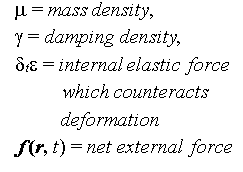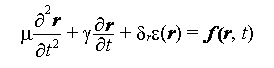where

The enery function for a deformable curve is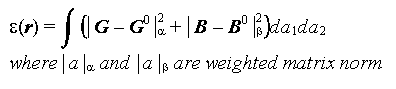The metric tensor G is defined as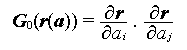The curvature tensor B is defined as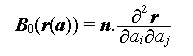where n is the unit surface normal vector at a

The differential form of the Lagrange Equation is discretised using a finite difference scheme. This gives a large set of simultaneous ordinary differential equations. Integrating these equations over time gives the postions, velocity, acceleration of all points. Using these we get a dynamic simulation of the cloth in the time period of interest. This model included the external force very effectively so both draping effects and waving effects could be done. Things like a waving flag, a carpet draped over different objects and tearing of cloth have been simulated excellently using this method.

3. The work of Sakaguchi4 - They developed a system called Party which simulated clothes not just cloth. The simulation was based on Newtons law of dynamics, that force on a body is propotional to the acceleration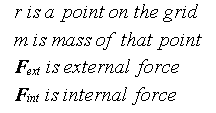where

Fext in most cases can be considered to be just the gravitational force downward. But it can be modified for modelling cloth in flowing air.

Fint = Fspring + Fviscous + Fplastic This is based on the Terzopoulos model.

They determined velocity and the postion of cloth using Euler's method. They also considered interactions of the cloth with the human body in terms of collisions including friction. They presented simulations that included a dress on a human body, cloth hanging from a pole and blowing in the wind.

4. The work of Provot5 - Provot used a very different approach for the physical model. This model was limited to cloth under constraints and did not include draping over solid objects. He modelled the cloth as a grid of springs. And classified the springs as flexion, structural and sheer springs as shown below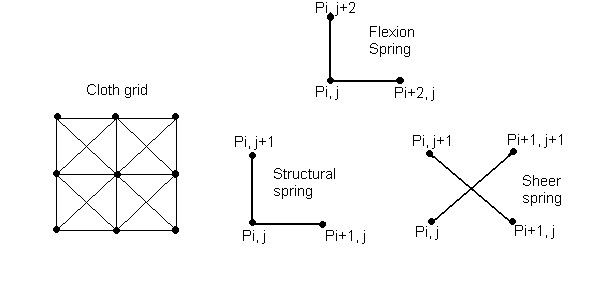He then used the Newtons law

Fext(i,j) + Fint(i,j) = m*acceleration(i,j)

Considering all the spring forces as the only internal forces present, he arrived at the expression for Fint(i,j) in terms of surrounding points as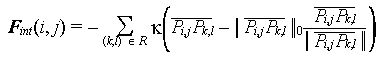where k is the stiffness of the springs, R is the set of 8 points surrounding P as shown in the figure, and,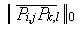is the undeformed length between the point P(i,j) and P(k,l)

Fext(i,j) was considered to consist of 3 components.

1. Fgrav(Pi,j) = mg,
where g is the acceleration due to gravity
2. Fdamp(Pi,j) = -cVi,j,
where c is the damping constant and Vi,j is the velocity of the point Pi,j
3. Fvisc(Pi,j)= q [ Ni,j . (Ufliud - Vi,j)] Ni,j
where q is the viscosity constant, Ni,j is the unit normal to the surface at the point Pi,j and Ufluid is the fluid velocity.

He then used the Euler method to integrate the Newton equation and obtain the postions and velocities at all instances of time. Here Provot observed the problem, that is common to structural mechanics that at the points of constraints the deformation goes to unrealistic values. To solve this problem, the stiffness can be increased, but this would increase the number of iterations required. So a bit of precalculation was done. He precalculated the deformation and if it exceeded a predetermined threshold then the elongation was arbitrarily limited to 10%.

We can see that this is a very comprehensive model, considering interactions with the between fluid and cloth not just inter-cloth interactions like other models. The damping term and viscosity term describe the fluid medium. Using this method we can model even cloth flowing in a water current!

5. The work of Ng6 - This team worked on similar lines to Feynman. But their work was more comprehensive with a better and faster energy minimization accompanied by a better multrigrid method. They were able to run many simulations like hanging cloth, cloth draped on a cube etc on a simple 486 machine (during 1995) in under 2 minutes! We wanted to deal extensively with this but could not get hold of the full paper as we couldnt contact the author.
6. Modelling the animation of motion of cloth 7 - They considered the particular situation of a piece of cloth immersed in airflow. The cloth is represented as a permeable stream surface in the form of mXn samll patches. They treated the problem using both fluid mechanics and structural mechanics. They used the simplified Reynolds averaged Navier Stokes equations to find the forces on each of these elementary patches and thus to determine distributed forces on the whole cloth. This gives the forces due to the fluid flowing. The structural cloth deformations are given by the Terzopoulos's model. Total cloth deformations are determined by vectorial addition of these two forces.

To find the forces due to the fluid on the cloth, they used many concepts which were primarily developed for aerodynamics. The real flow is nonlinear and complicated. But for slow air velocities we can assume the flow to be incompressible, irrotational and inviscid. This simplifies thing to a large extent. Most importantly the flow on the cloth can be described in terms of a potential function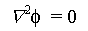, . The velocites are then given by the equation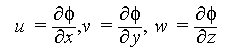The force is found indirectly using the pressure. The pressure is related to the velocity by the Bernoulli equation given by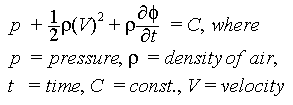All we have to do is solve the potential function for the given boundary conditions.

The cloth is divided into mXn patches. To find the exact potential for the whole cloth, we simulate it by the superposition of vortex fields placed at each patch. The potential of a vortex is a standard result. So our final potential is the sum of all the potentials, which means we can get the velocities and then the pressure (which gives the force). The advantage in using potentials is that it is a scalar and we can add up all potential fields for the patches of cloth.

To find the actual value of the vortex field at every patch, we need the boundary conditions.

• One condition is that at infinity the presence of the cloth does not make a difference to the flow, ie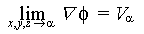.
• At the cloth surface, the boundary condition is that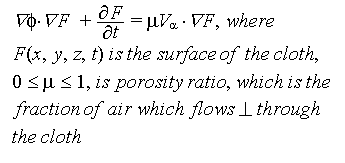.
• Another condition is the so called Kutta condition. In aerodynamics this is a condition which is applied at the trailing edge of the wing. It state that

Flow leaves the trailing edge of the wing smoothly.

It is a condition which cannot be proved but is always used. More details require quite a bit of fluid mechanics. So we will not deal with those details.

Interested readers can refer to J D Anderson - Fundamentals of Aerodynamics or Panton - Incompressible Flows. We are suggesting these two books, because those are ones we have used on our courses. I am sure any book on aerodynamics will cover these topics. We are not dealing wih this model very much in detail because it requires quite a bit of fluid mechanics knowledge. This being a computer science tutorial most of the target audience may not have much of those fundas. Interested reader can look at the full paper which is quite thorough and understandable easily.

At the trailing edge of the cloth we enforce this condition. We can solve for the vortex field at each patch of the cloth, which will ultimately lead us to the pressure and force at each patch.

In this model cloth placed near walls can also be modelled by using a simple technique called the method of images. Essentially what it means is that, whenever we have a wall, we think of it as a mirror. Then for a vortex at a point, at the same distance behind the mirror (wall) we place a vortex of opposite strength (this vortex is called the image). This will result in zero flow at the wall surface, which is the physical effect we will get in reality due to the presence of a wall. Our potentail will now include the potential due to vortex fields of the cloth and the extra image vortex fields we have placed "behing the wall".

The reported simulations used 200 patches to represent the cloth and required about 3 seconds on a SGI Power workstation to complete a 1000, step calculation of the distributed forces. The models memory requirement will increase enoromously if larger numbers of patches are used.

They initially treated the airflow as quasi-steady, that is the instantaneous airflow velocity is unchganed with time. This simplifies things to a very large extent in the sense the Fluid forces are very easy to calculate. They later modified it to treat the airflow as unsteady ie constant change of air velocity. The simulation time is obviously longer for the second version. it took about 32 seconds for the same peice of cloth. They produced a flag blowing in the wind which was very impressive.

## A Particle Based Model for Cloth draping 8

The drape of woven cloth has intrigued humans for centuries. This is evident in the flowing robes contained in the sculptures of ancient Rome, the intricate folds of fabric depicted in the paintings of the Renaissance, and the elaborate billowing clothing of the 18th century. Even in the modern times artists such as Christo realise that the image of draping cloth over a structure like the Reichstag in Berlin is fascinating and provocative. It has always been clear that woven materials have unique properties that allow them to deform in ways significantly different than other sheet materials, eg paper, vinyl and metal foils. Cloth's special deformation capabilities have been noted and recognized through the ages but never fully understood from scientific or engineering perspective.

### Introduction

We will try to develop a new technique for reliably reproducing the chracteristic draping behaviour of particular fabrics. We will try to make fabric dependent simulations. We will try to answer questions like "Will this cloth drape more nicely when it is made of silk rather than cotton?" etc. The model uses the Kawabata fabric measurement system to differentiate between cloth fabrics.

To date most of the efforts to create a model of cloth have employed continuum mechanics, with simulation utilizinng finite element or finite difference methods. They did not consider the integral particle nature. There is a subtle difference between integral particle nature and solving the continuum mechanics problem by discretising. At first glance both seem to be the same. The difference is that in the continuum mechanics approach we define a behaviour of the cloth which is exhibited at the macroscopic level and then discretise the problem and assume that only these macroscopic interactions are present at this level. This becomes very wrong when we consider cloth because of the inherent microscopic interactions of friction between weaves.

Another continuum approach has been to analyse cloth at the microscopic level and then make statistical assumptions about the spread of these micorscopic interaction at the macroscopic level. This leads to a definite and large loss of data.

Cloth has defied all these attempts by textile scientists to make an accurate model. The problem is that the assumptions on which these techniques are based, that the behaviour of a small element is governed by the same continuum equations that describe the large-scale behaviour, are not valid for woven materials. In fact, cloth is a complex mechanism whose important mechanical elements are at a scale that is not far from the scale of the mesh element that would be used in a typical simulation. The mesh size cannot be reduced further even if we harness lots of computational resources, because the thread size itself is the height of minuteness! In cloth, fine fibers are spun into yarns or threads and these threads are more or les tightly woven into an interlocking network. The network varies from fabric to fabric. All these components are held together not by molecualar bonds or welds, but just by friction. Behaviour depends on type of fiber(cotton, silk, wool etc), weight of the yarn, tightness of weave, type of weave etc.

This model is an application of interacting-particle techniques. We attempt to capture low-level microscopic interactions within a material, and is founded on the premise that by modelling the low-level structures of a material and computationally aggregrating their interactions, correct macroscopic behaviour will emerge.

Such studies based on extending microscopic behaviour to get the macroscopic behaviour have been done in other fields. Some of them are given below.

• Reeves and Blaui used this concept to create trees and grass.
• Simsii demostrated how particle systems could be used on a massivel parallel computer to simulate things such as waterfalls, explosions, vortex fields etc.
• Reynoldsiii developed a method which could be used to determine the herding and flocking of animals. The method was based on interacting particles.

### Capturing Cloth Microstructure with Energy Functions

In this model we will attempt to express the various interactions between the cloth particles at the microscopic level. It is neither diserable nor computationally practical to represent the full details of the underlying thread structure of woven fabric, but we can try to spot out the most important interactions. We model cloth as a set of particles that are located at the crossing points of the warp and weft threads in the cloth as in figure below.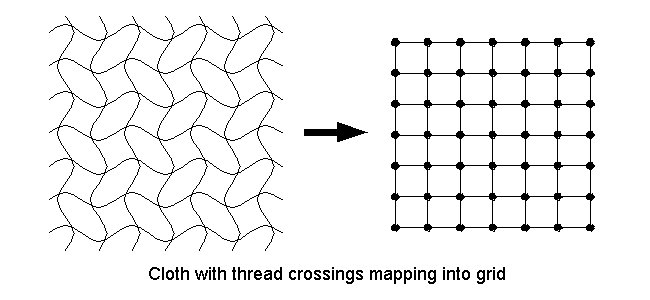Several of the most prominent interactions occur at these points. Most importantly and conveniently for us, the compression force amongst the weaves is so great that the threads are effectively clamped at these points providing an axis for bending. Other interactions such as stretching can be expressed as the displacement of the particle that we have defined.

In this model, we represent the various constraints and interactions occuring at the thread level with energy functions that capture simple geometric relationships between particles within a local neighbourhood. The basic interactions that occur at the thread level, which are important are:

• Contact
• Stretching
• Bending
• Trellising - this is the most queer of the interactions

The total energy can then be defined as

Utotal = Urepel + Ustretch + Ubend + Utrellis + Ugravity

The origin of the different energy functions is given below. This is just a physical explanation to give you a feel of the energies. A detail mathematical derivation follows later.
 URepel UStretch UBend UTrellis UGravity It is an artificial energy of repulsion which we imagine, to enforce the condition that there is a minimum distance between particle preventing cloth self-intersection. Energy that connects each particle to its 4 neighbours and represents the tensile strain. Energy due to threads bending out of the immediate plane of the surrounding cloth Energy due to bending around a thread crossing in the plane. The thread forms an S shape due to this. At a macroscopic level this is the shear produced in the cloth. Graviatational potential energy due to concentrated mass of particle

We will now deal with energy term and derive a mathematical formula for each.

### Repelling and Stretching

The Kawabata system defines the tensile strain data for all fabrics. But we have chosen to ignore it because when a cloth is draping under its own weight the strain it suffers is quite negligible. So we do not have any cloth-specific functions for these energies. The stretching and repelling functions together provide a steep energy well that acts to keep 4-connected neighbour particles at a nominal distance w (this is what prevents cloth self intersection). By hand sketching the curves as shown in the figure below we can use the functions given by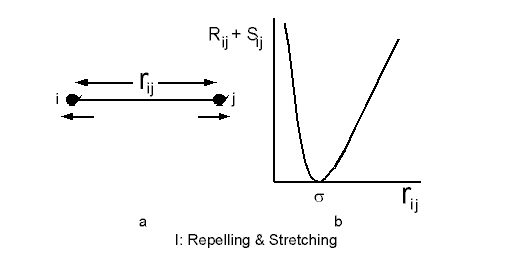R(ri,j) = C[(w - rij)5/rij] ri,j <= w 0 ri,j > w
 S(ri,j) = 0 ri,j <= w C[((rij - w)/w)5] ri,j > w

where C is the scale parameter which sets the magnitude of the repulsion and stretching forces.

Please note that here i,j are not the perpendicular axis coordinates. i, j are 2 successive points.

For a point i, Urepeli is calculated by summing over all points of the cloth, which can be expressed as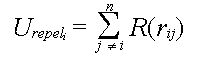In practice we dont have to sum over the whole domain of points as the function will die out to 0 values at points which are not nearby (this follows from the definition for distances > w).

An energy well is produced by directly connecting each point with the stretching potential to its 4 neighbouts. It is calculated by summing S for each neighbour as given by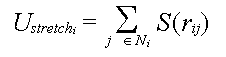where Ni is the set of four-connected neighbours to particle i. The combined repelling and stretching functions enforce the distance constraint between neighbouring particles.

### Gravity

The particle energy due to gravity is defined as

Ugravityi = mighi
where mi is the mass, hi is the height of the particle and g is the acceleration due to gravity. While choosing the mass we have to take the concentrated mass of the cloth at that point.

### Bending and Trellising

In contrast to the stretching and repelling, the bending and trellising properties are significant even when a cloth drapes under self-weight. Therefore for a cloth specific draping behaviour these energy functions have to be designed based on the Kawabata system.

Bending of the thread is shown in the figure below. It is a function of the angle formed by the 3 particles along a weft or warp. The figure below gives the angles along a weft. Just rotate by 90 to visualise angle along a weft.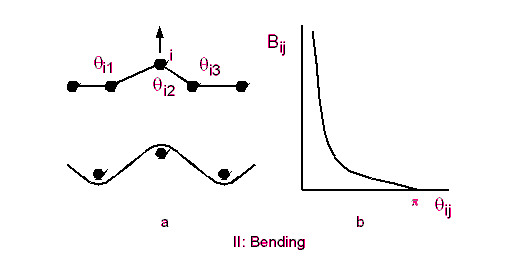The total bending energy is then given by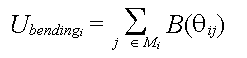where Mi is the set of six angles formed by the segments connecting i to the nearest neighbours along the horizontal and vertical. The 3 angles along the horizontal have been shown in the figure.

Trellising is shown in the figure below. In the graph, two segments are formed by joining the nearest horizontal and vertical neighbours. An equillirium angle between them is then 90. When the cloth drapes, this will change and give an S shape as shown. We have shown only trellising in one direction. The trellis angle is the angle formed between the equillibrium line segment and the line segment joining the current location of the points. The figure below explains it clearly.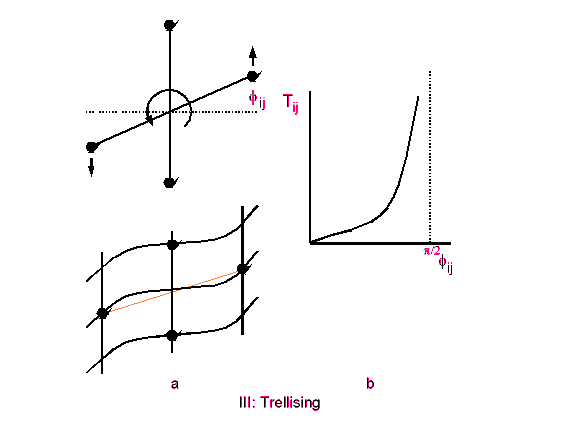The trellis energy then is summed for 4 angles for every point. It can be written as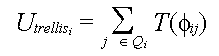where Ki is the set of four trellising angles formed by the 4 points.

### Initial Implementation

The energy curves we have drawn are just guesses. But simulations can be done using this and give very satisfying results, proving that the energy functions we have obtained are the important and signficant ones.

The simulation is done in two steps.

1. Modelling the effect of gravity alone and the geometric constraint of the object on which the cloth is draping.
2. Mimimizing the potential energy term we have obtained to get the final shape of the draped cloth.

This technique coupled with the sketched in energy curves produced satisfying results as reported by Breen et al in their paper. Their simulation figure is reproduced below.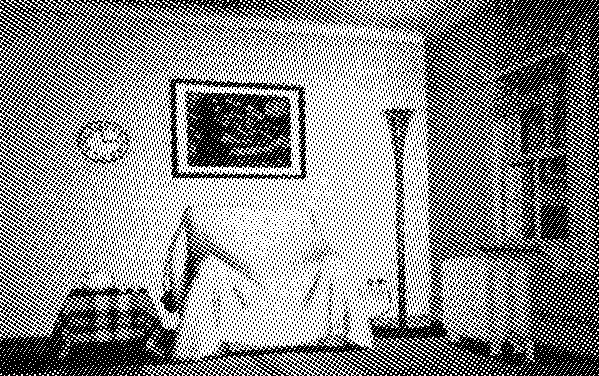The drawbacks of this initial model.

1. It was not based on any physical units and so we cannot determine the actual size of the simulated cloth.
2. The cloth cannot be queried for any physical information.
3. Particular kinds of cloth cannot be simulated.

### Minimizing the energy

In their original paper Breen et al used a very complicated Stochastic Gradient Descent for minimizing the energy. They used this because the simulation they did was not custom coded. They did it on an object oriented RS6000 system. Their simulation times were in the order of days! This does not mean the method is computationally impractical.

We will discuss a much simpler and customizable minimization technique using just the first order derivative.

First of all, we rewrite the energy expression in terms of the coordinates of a point (x, y, z). We can determine the angles in terms of the relations between coordinates of the neighbours. Ultimately we will have the whole energy equation in terms of coordinates as given below.

U = f(x, y, z)

Urepel and Ustretch are be functions of rij, where rij is the distance between points i, j.

rij = sqrt[ (xi - xj)2 + (yi - yj)2 + (zi - zj)2 ]

For bending we need the angles in terms of the coordinate of the points. This can be written very simply in terms of cross products of vectors. Refer to figure for bending and trellising. For a point i, we define ri,i-1 and ri-1, i-2 as the vectors connecting the points denoted by their subscripts. Then

thetai1 = cos-1 ( ri,i-1 . ri-1,i-2)

Similarly we can express the other angles for bending and trellising.

We now have the energy in terms of coordinates (x, y, z) of each particle. For minimum energy,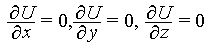Differentiating the energy with respect x, y and z, and using only the first order terms, we get simultaneous equations envolving the grid elements. Remember here that the grid elements are numbered serially and have a single subscript i.

Example:
For conciseness let us assume the energy term is exclusively made of the stretching energy, and the function S = rij2
where

rij = sqrt[ (xi - xj)2 + (yi - yj)2 + (zi - zj)2 ]

For a point i we need to sum this for 4 surrounding points. We number the points serially with a 50 X 50 grid. Then the points are i-1, i+1, i+50, i-50. We get

U = [ (xi - xi-1)2 + (yi - yi-1)2 + (zi - zi-1)2 ] +
[ (xi - xi+1)2 + (yi - yi+1)2 + (zi - zi+1)2 ] +
[ (xi - xi+50)2 + (yi - yi+50)2 + (zi - zi+50)2 ] +
[ (xi - xi-50)2 + (yi - yi-50)2 + (zi - zi-50)2 ] +

We now differentiate this with respect to x, y, z. Considering the x term give us
xi - xi-1 - (xi-1 - xi-2) +
xi - xi-1 - (xi+1 - xi) +
xi - xi-1 - (xi+50 - xi+50-1) +
xi - xi-1 - (xi-50 - xi-50-1) = 0

Considering all 50 * 50 points we get that many simultaneous equations, which when solved will give the solution for location of all points. The boundary condition is given by the geometric shape of the object on which the cloth is draping.

If you couldnt follow the math above, just try to derive the minimization process yourself and you will be able to figure it out.

### Cloth specific tuning of the model

In order to give our model a solid physical grounding, we develop the energy equations from the Kawabata Evaluation System. This is a standard equipment to measure physical and mechanical properties of cloth. By basing the energy equations on this, our model becomes truly physical and quantitative. We can then measure mechanical properties of a particular woven material and simulate the exact draping behaviour.

The following section requires some knowledge of structural mechanics like bending moment, shear force, theory of beams etc. The final aim is to get functions B and T that give the bending and trellising energies. You can just pick the final results if you want, which are given in a table at the end. Click here to see table.

The Kawabata System may be used to measure the bending, shearing and tensile properties of cloth, in addition to its surface roughness and compressibility. For bending, shearing and tensile properties, the equipment measures how much force is required to perform three kinds of deformation on a fabric sample of standard size and shape. The system produces plots of each force as a function of come geometric parameter like (angle, length etc).

The exact procedure is painfully mathematically involving curve fitting etc. It makes no sense to discuss the exact procedure of the Kawabata System. Please refer to the original paper for full details. We will assume that we know the final equations of the curve. A sample Kawabata plot for 100% cotton for shear is reproduced below.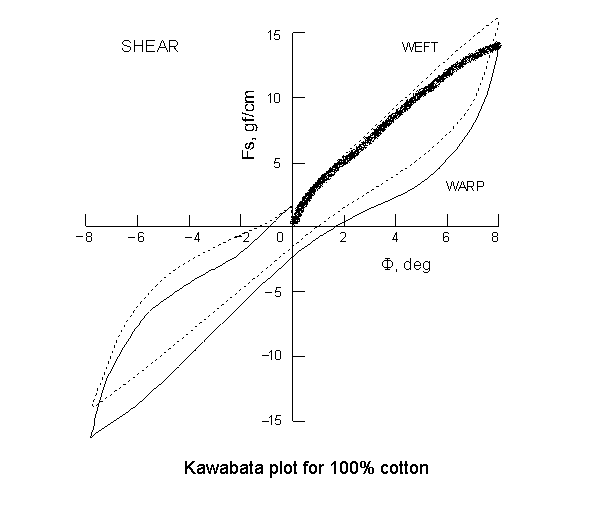The curve gives the variation of shearangle with shear force. The curve is plotted by slowly increasing the shear force in one direction, noting down the shear angle, then slowly decreasing it and noting down the angel. Then we repeat the process applying the shear force in the opposite direction. This will naturally produce hysterisis in the cloth. We will ignore this hysterisis and assume that the same path is followed while increasing and decreasing and in both directions. So we have to find the equation of only the curve that is marked by thickening in the figure.

Our aim is to describe this curve using peicewise continuous polynomials so that we can read off one value from the graph when the other value is known. We will require this to define our trellising and bending energies.

When we are done with formulating the Kawabata curve in terms of peice-wise polynomials we need to relate the energy equation to this curve. In the case of bending, the curves relate bending moment to curvature. In the case of trellising, the curves relate force to the shear angle. We need energy as functions of the bending and trellising angles available.

#### Bending Energy Equations

Within the low deformation region within an individual thread, we assume that the theory of elastic bending beams is applicable, and can be used to calculate energy of bending. The strain energy dU due to bending stored in a segment dS of beam is given by

 dU = MdS 2R

where M is the bending moment acting on the segment, R is the radius of curvature, which is related to curvature by K = 1/R.

In our model, each particle is seperated from its 4-connected neighbouring particles by the equillibrium distance w. Therefore each particle represents a w X w peice of cloth. This peice of cloth can be considered as beams in parallel. Then the length of a beam is w. For one beam the energy becomes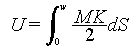If we assume that over the length of the thread in the w X w patch the bending moment and the curvature are constant we get,

 U = MK w 2

M is given in moment per unit length, so the energy for all the threads in the square peice of cloth is directly,

 B = MK w2 2

We know M in terms of K from the Kawabata plot. Finally the Kawabata plot is used! But we still need K the curvature. Curvature at a point can be considered to constant between its 2 neighbours. Then we need to fit a circle between the 2 neighbours of a point. Refer to the bending and trellising figure. We get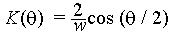As the angle goes to zero, K also goes to a finite number 2/w. This is not what we physically expect, because as the angle goes to zero the thread bends on itself, giving a curvature of infinity. To rectify this anomaly we modify the curvature such that it goes to infinity as the angle goes to 0. So we arbitrarily assume the above equation only for theata lying between 180 and 45. We then fit the curve a/x + b to the postion and slope at 45 due the above equation. So we get the complete curvature.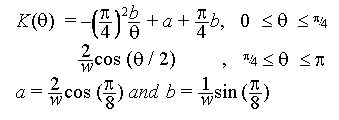we get a and b by equating the a/theta + b to our previous equaition at 45 to obtain and continuity and differentiability at all points.

We now apply the same logic for the vertical thread to obtain a similar equation for warp.

#### Trellising Energy Equations

Producing the trellising energy equations is a bit simpler than the bending energy equations. The work dW produced by a force F acting over a displacement dS is,

dW = FdS

If we assume that the width l of the sample remains constant during shearing, then the path travelled by the point at which the shearing force is applied is a circular arc whose length is defined by S = lA, where A is the shearing angle. From this relationship, it can be deduced that dS = ldA. If the force point is moving along a circular arc, its direction is not parallel to the direction of force. Fcos(A) is the component of force along the direction of displacement. We can then derive the total energy for shearing as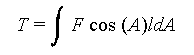For a specific material, we can use the Kawabata curves for shearing force as a function of A, and integrate to get the total energy T.

We have defined the Kawabata curve only for small deformations (typically to a maximum of 10degrees). For large deformations we need to define the Kawabata curve intuitively. Most woven materails do not shear more than 45o. To be on the safer side we assume a maximum of shear of 60o. At this point our shear force must go to infinity. So we fit the curve

a/(60o - A) + b

to the slope and position of the endpoint of our Kawabata curve at around 10o.

We are almost at the end of the road. Just before we discuss results and simulations that have been produced using this method we will list out what we have done.

1. Defined the internal energy of the cloth at the particle level.
2. Derived approximate energy curves by guessing to simulate "general cloth".
3. Drawn the Kawabata plots for diffferent cloth and expressed the plot as peicewise polynomials.
4. Derived a rigorous energy equation based on strucural mechanics.
5. Used this data in the energy equations to develop cloth specific energy equation.
Bending Energy Trellising Energywhere A is the trellising angle, and F is given as a function of the trellising angle in the Kawabata curve for shear.
 B = MK w2 2
M is given as a function of K in the Kawabata curve for bending.

### Experimental Results

We have derived energy equations and using these we should able to predict the behaviour of cloth. We can make our own Kawabata plots now. We will not be presenting these curves here. We can also actually drape different cloth on different objects and *see* the effects. In their original paper the actual results were produced by Breens team. We were unable to get the exact images and reproduce them in this paper.

They created images of the draping on a cube and took actual photos when that type of cloth is draped on the cube. There was a striking similarity between the two, validating our struggle till now!

There obviously are differences between the two images. But the reason as proposed by Breen and his team is that:

Certainly the cloth simulations do no exactly produce the actual draping configurations. This, in a sense, would be impossible, considering the chaotic and stochastic nature of draping a cloth over an object. Since a table cloth will never fall in exactly the same way every time it is draped, it is unrealistic to expect that a stochastic simulation would be able to precisely reproduce any one draping configuration!(exclamation ours)

But we can make some clear conclusions from the drapings.

1. The stiffness of cotton ensured that it would produce a single draping structure at the corners.
2. The polyester/cotton blend always produced a kind of asymetric fold that would wrap around the corners.
3. Wool either forms a single fold or collapses into many folds.
A glaring difference is that the simulated draping seemed to produce soft corners, but the real ones had sharp corners. This is because the simulated draping was done with a grid of 50x50. In such a grid the sharpness of the bends and edges cannot be captured.

## Conclusion

We have discussed Geometric modelling of cloth which is the easiest, some physical models which considered cloth as one deformable object, and finally a very interesting particle based method to predict the draping behaviour of cloth. You should be able to write your own code and make your own draping simulations. There are many applications of all this cloth modelling like interior design, modelling dresses for virtual actors etc.

Current cloth visualization techniques lack the means for fashion designers to input their requirements. Models should help designers visualize their ideas during the garmnet design process. Before a garment is manufactured, fashions designer already have a concept of what it will look like when it is worn. They have expressed their designs with pencil sketches. They should be able to move this on to computers and simulate the whole finished product and check out without manufacturing a single peice all the properties of the finished product. We are still far away from this goal. We hope this tutorial has explained to you salient features of cloth visualization and you will help in reaching that goal.

## Glossary

Kawabata System
This is a system of curves and relations expressing the physical behaviour of fabrics used to classify and capture the essential qualities of different fabrics. Cloth specific simulations are based on this system. Examples are plots of shear angle with shear, curvature with bending moment etc.
Trellising
This is the effect of the threads moving in such a way to form an S. The macroscopic effect is exhibited as shear. Refer to figure.
Warp
This is the threads in the horizontal directions
Weft
This is the threads in the vertical directions

## References directly related to cloth modelling

1. J. Weil, The Synthesis of Cloth Objects, Computer Graphics, (Proc SIGGRAPH), Vol 20, No 4, 1986
2. C. Feynmann, Modelling the Appearance of Cloth, masters thesis, Dept of EECS, Massachusetts Inst. of Technology, Cambridge, 1986
3. D. Terzopoulos et al, Elastically Deformable Models, Computer Graphics (Proc SIGGRAPH), Vol 21, No 4, 1987
4. Y. Sakaguchi et al, PARTY: Physical Environment of Artificial Reality for Dress Simulation - A Dynamically Deformabel Model of Dress, Trans. Soc. of Electronics, Information and Communications, Dec 1991
5. X. Provot, Deformation Constraints in a Mass-spring Model to Describe Rigid Cloth Behaviour, Proc of Graphics Interface, 1995
6. H.N. Ng et al, A System for Modelling and Visualization of Cloth Materials, Computers and Graphics, Vol 19, No 3, 1995
7. Li Ling et al, A Model for Animating the Motion of Cloth, International Journal of Systems and Applications in Computer Graphics, November 1994
8. David Breen et al, A Particle-Based Model for Simulating the Draping Behaviour of Woven Cloth, Textile Research Journal, Vol 64, No 11, 1994
9. Bernhard Eberhardt et al, A Fast, Flexible, Particle-System Model for Cloth Draping, IEEE Computer Graphics and Applications, September 1996

## Other references

1. Reeves, W.T. and R. Blau, Approximate and Probabilistic Algorithms for Shading and Rendering Structured Particle Systems, Computer Graphics (Proc SIGGRAPH), Vol 19, No 3, 1985
2. Sims K, Particle Animation and Rendering Using Data Parallel Computation, Computer Graphics (Proc SIGGRAPH), Vol 24, No 4, 1990
3. Reynolds C.W., Flocks, Herds and Schools: A Distributed Behavioral Model, Computer Graphics (Proc SIGGRAPH), Vol 21, No 4, 1987

## Appendix

Thix appendix consists of

### Source Code

Build using Turbo C++. For any doubts or clarifications, please contact authors at [email protected]

To use the source, do not cut the HTML from here, < and > signs have been replaced with their HTML escape codes. Go to your web browser and use the Save as text option of your web browser.

```#include <stdio.h>
#include <conio.h>
#include <math.h>

int main(void)
{
float y, x;
float grid;  /* cloth grid */
int i, j, k;
FILE *f;

/* initialise grid to 0 */
for (j = 1; j <= 24; j++) {
for (i = 1; i <= 24; i++) {
grid[i][j] = 0;
}
}

/* generate catenary. assume length of cloth to be 12 units. So
curve runs from -6 to +6. Assume a step of 0.5.
So we get a grid of 24 points in size
Square cloth hanging from ceiling. So all edges have same value.
*/
i = 0;
for (x = -6.0; x <= 6.0; x=x+0.5) {
y = 100*cosh(x/10);
putpixel(320+x*10, 240 - y, WHITE);
gotoxy(1,1);
printf("%3.2f, %3.2f", x, y);
grid[i] = y;   /* left */
grid[i] = y;  /* right */
grid[i] = y;   /* bottom */
grid[i] = y;  /* top */
i++;
}

getch();

/* do an arbitrary iteration of 100 steps to converge to final value */
for (k = 0; k <= 100; k++) {
for (j = 1; j <= 23; j++) {
for (i = 1; i <= 23; i++) {
grid[i][j] = ( grid[i-1][j] + grid[i+1][j] + grid[i][j-1] + grid[i][j+1] ) / 4;
}
}
}

/* output data to  file.*/
/* format of file:

file consists of a set of polygons in 3D.
Each polygon consists of 4 vertices.

first line - no of vertices.
vertices

example

4
-1.2000 0.0000 1.1855
-1.1000 0.0000 1.1551
-1.1000 0.1000 1.1289
-1.2000 0.1000 1.1551
4
-1.1000 0.0000 1.1551
-1.0000 0.0000 1.1276
-1.0000 0.1000 1.1029
-1.1000 0.1000 1.1289

*/
f = fopen("cloth.j3d", "wt");
for (j = 0; j <= 23; j++) {
for (i = 0; i <= 23; i++) {
fprintf(f, "4\n");
fprintf(f, "%3.4f %3.4f %3.4f\n", (i-12)/10.0, j/10.0, grid[i][j]/100);
fprintf(f, "%3.4f %3.4f %3.4f \n", (i-12+1)/10.0, j/10.0, grid[i+1][j]/100);
fprintf(f, "%3.4f %3.4f %3.4f \n", (i-12+1)/10.0, (j+1)/10.0, grid[i+1][j+1]/100);
fprintf(f, "%3.4f %3.4f %3.4f \n", (i-12)/10.0, (j+1)/10.0, grid[i][j+1]/100);
}
}
/* we divide x, y coord by 10, z(height) coord by 100 to scale the image
to give us a nice image */
fclose(f);
printf("Completed. Press any key to exit.")
getch();
}
```
;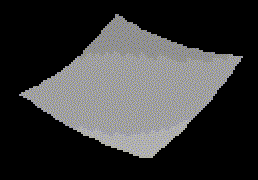The above code generates a set of polygons which are assumed to form a surface. The image here was generating by raytracing the surface, with the diffusion effects of light, which is why the shading effects are also visible.

Last updated on May 2, 1998.Get your own Free Home Page